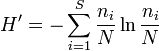# How to calculate species abundance and diversity

• blicker
In summary, the individual tried to solve a problem they weren't studying and didn't know how to solve it. They found an article that explained the concept.

## Homework Statement

This is the sample population taken along a 5m transect:
ants:9
grasshoppers:1
pill bugs:4
spiders:1

## Homework Equations

What formulas would i use to calculate the species abundance and diversity?

## The Attempt at a Solution

I only know this formula H'=-$\Sigma$ (n$_{}i$/N)xIn(n$_{}i$/N) and i don't know what to do with this formula.

Hi blicker!Can it be that your formula is actually:where "ln" is the natural logarithm (and also a button on your calculator), and where ni is the number of individuals of species i?

See: http://en.wikipedia.org/wiki/Shannon_index

yes that's it! but i don't know what numbers go where or how to calculate it. like would i include the sigma symbol and the letters above and below it?

The sigma symbol indicates that you have to sum.
So:
$$H'=-\sum_{i=1}^S {n_i \over N} \ln {n_i \over N} = -({n_1 \over N} \ln {n_1 \over N} + {n_2 \over N} \ln {n_2 \over N} + {n_3 \over N} \ln {n_3 \over N} + {n_4 \over N} \ln {n_4 \over N})$$

From wiki:
* ni The number of individuals in species i; the abundance of species i.
* S The number of species. Also called species richness.
* N The total number of all individuals
* pi The relative abundance of each species, calculated as the proportion of individuals of a given species to the total number of individuals in the community: $n_i\over N$

so would the equation for ants look like this:
-(9/15)In(9/15)

blicker said:
so would the equation for ants look like this:
-(9/15)In(9/15)

Well, Shannon's index specifies to sum all the terms.
So what you mention is only part of H'.

Btw, it is $ln$ ("logarithmus naturalis").

And apparently $n_i$ is the "species abundance".
Furthermore $p_i={n_i \over N}$ is the "relative species abundance".